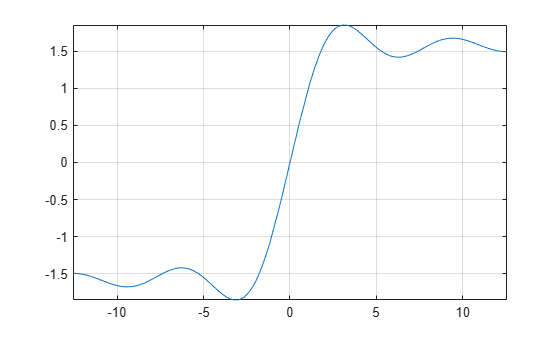# sinint

Sine integral function

## Syntax

``sinint(X)``

## Description

example

````sinint(X)` returns the sine integral function of `X`.```

## Examples

### Sine Integral Function for Numeric and Symbolic Arguments

Depending on its arguments, `sinint` returns floating-point or exact symbolic results.

Compute the sine integral function for these numbers. Because these numbers are not symbolic objects, `sinint` returns floating-point results.

`A = sinint([- pi, 0, pi/2, pi, 1])`
```A = -1.8519 0 1.3708 1.8519 0.9461```

Compute the sine integral function for the numbers converted to symbolic objects. For many symbolic (exact) numbers, `sinint` returns unresolved symbolic calls.

`symA = sinint(sym([- pi, 0, pi/2, pi, 1]))`
```symA = [ -sinint(pi), 0, sinint(pi/2), sinint(pi), sinint(1)]```

Use `vpa` to approximate symbolic results with floating-point numbers:

`vpa(symA)`
```ans = [ -1.851937051982466170361053370158,... 0,... 1.3707621681544884800696782883816,... 1.851937051982466170361053370158,... 0.94608307036718301494135331382318]```

### Plot Sine Integral Function

Plot the sine integral function on the interval from `-4*pi` to `4*pi`.

```syms x fplot(sinint(x),[-4*pi 4*pi]) grid on```### Handle Expressions Containing Sine Integral Function

Many functions, such as `diff`, `int`, and `taylor`, can handle expressions containing `sinint`.

Find the first and second derivatives of the sine integral function:

```syms x diff(sinint(x), x) diff(sinint(x), x, x)```
```ans = sin(x)/x ans = cos(x)/x - sin(x)/x^2```

Find the indefinite integral of the sine integral function:

`int(sinint(x), x)`
```ans = cos(x) + x*sinint(x)```

Find the Taylor series expansion of `sinint(x)`:

`taylor(sinint(x), x)`
```ans = x^5/600 - x^3/18 + x```

## Input Arguments

collapse all

Input, specified as a symbolic number, variable, expression, or function, or as a vector or matrix of symbolic numbers, variables, expressions, or functions.

`$\text{Si}\left(x\right)=\underset{0}{\overset{x}{\int }}\frac{\mathrm{sin}\left(t\right)}{t}dt$`